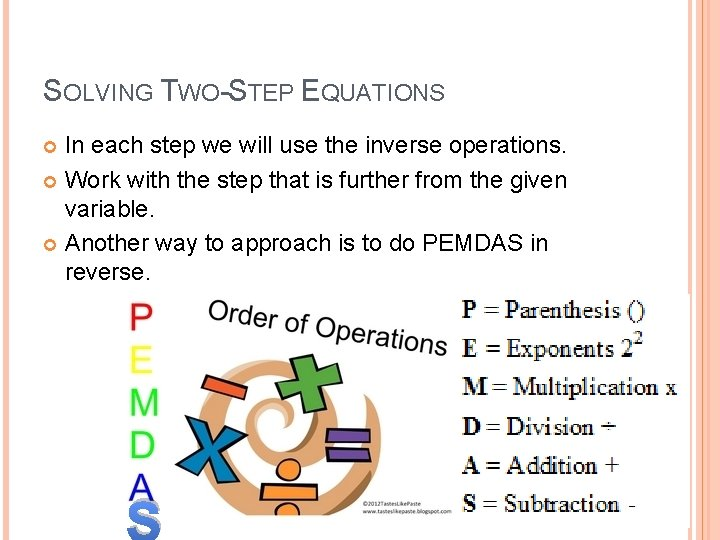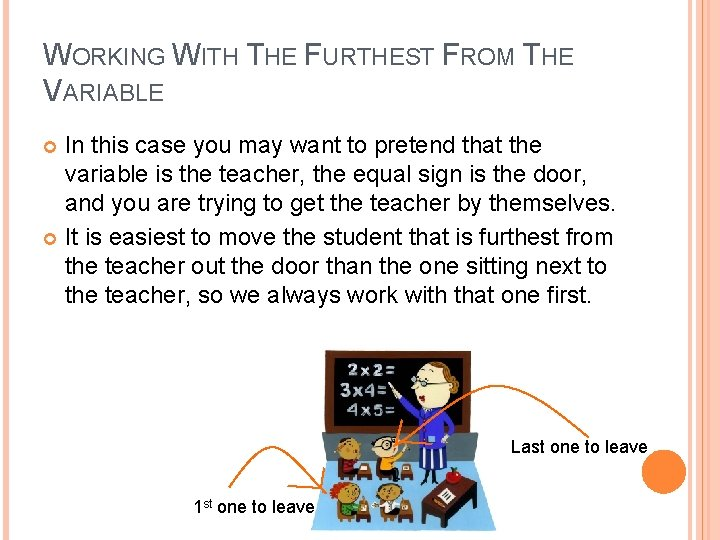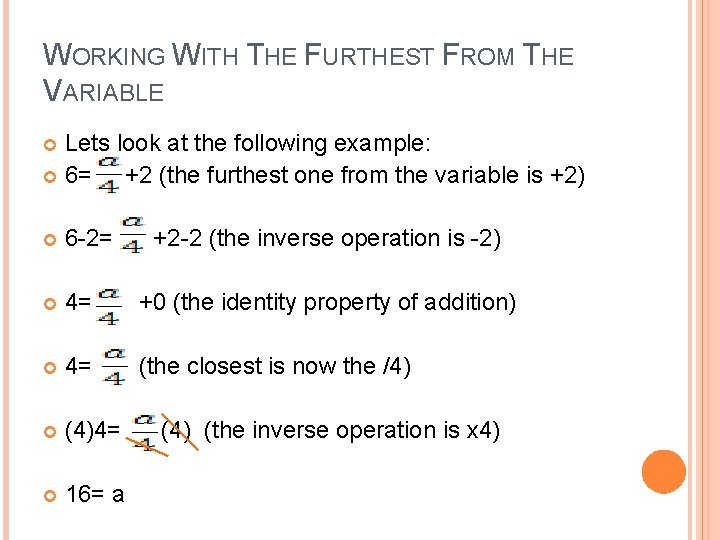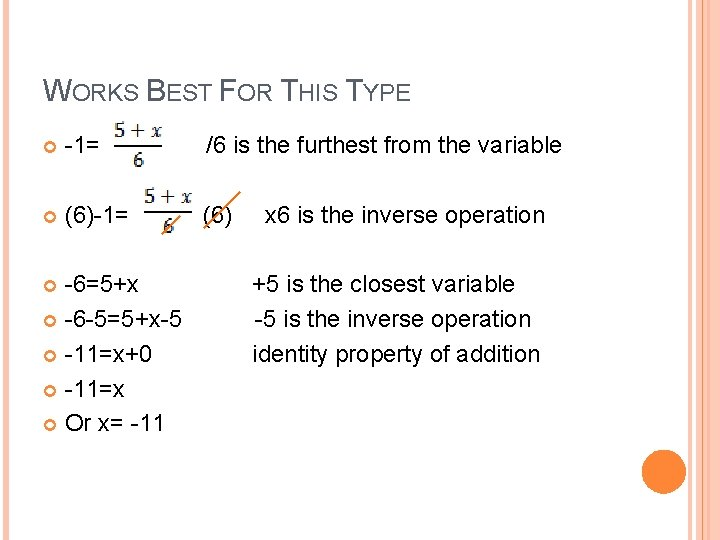# SOLVING TWOSTEP EQUATIONS By Mrs Muller SOLVING TWOSTEP

• Slides: 6SOLVING TWO-STEP EQUATIONS By Mrs. MullerSOLVING TWO-STEP EQUATIONS In each step we will use the inverse operations. Work with the step that is further from the given variable. Another way to approach is to do PEMDAS in reverse. SWORKING WITH THE FURTHEST FROM THE VARIABLE In this case you may want to pretend that the variable is the teacher, the equal sign is the door, and you are trying to get the teacher by themselves. It is easiest to move the student that is furthest from the teacher out the door than the one sitting next to the teacher, so we always work with that one first. Last one to leave 1 st one to leaveWORKING WITH THE FURTHEST FROM THE VARIABLE Lets look at the following example: 6= +2 (the furthest one from the variable is +2) 6 -2= +2 -2 (the inverse operation is -2) 4= +0 (the identity property of addition) 4= (the closest is now the /4) (4)4= 16= a (4) (the inverse operation is x 4)WORKS BEST FOR THIS TYPE -1= /6 is the furthest from the variable (6)-1= (6) -6=5+x -6 -5=5+x-5 -11=x+0 -11=x Or x= -11 x 6 is the inverse operation +5 is the closest variable -5 is the inverse operation identity property of additionPEMDAS IN REVERSE In this case we work with PEMDAS in reverse: SADMEP Let’s look at the following example: -9 x+1= -80 we start with addition/subtraction -9 x+1 -1= -80 -1 the inverse operation of +1 is -1 -9 x+0= -81 the identity property of addition -9 x= -81 the next step is multiplication/division -9 x/-9= -81/-9 the inverse operation of -9 x is /-9 X=9